Скачать презентацию 1 Compound 9 Interest Compound Chapter 9 Mc

f82c6be72ef74bff421d7c22df691f8d.ppt

• Количество слайдов: 57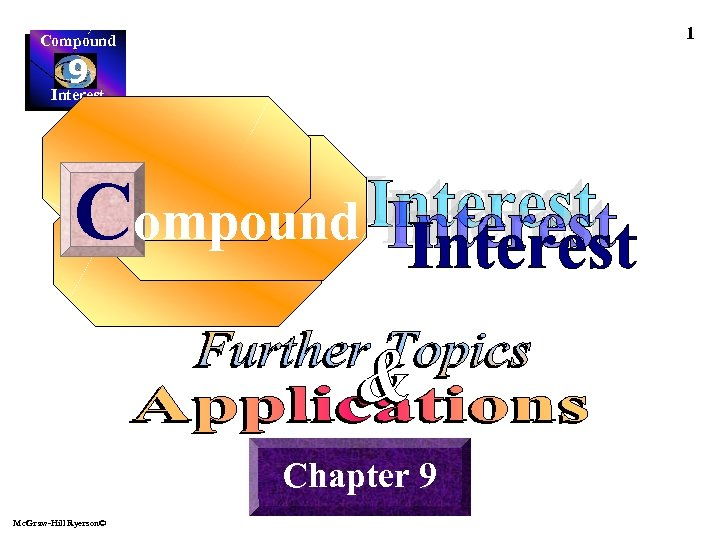1 Compound 9 Interest Compound Chapter 9 Mc. Graw-Hill Ryerson©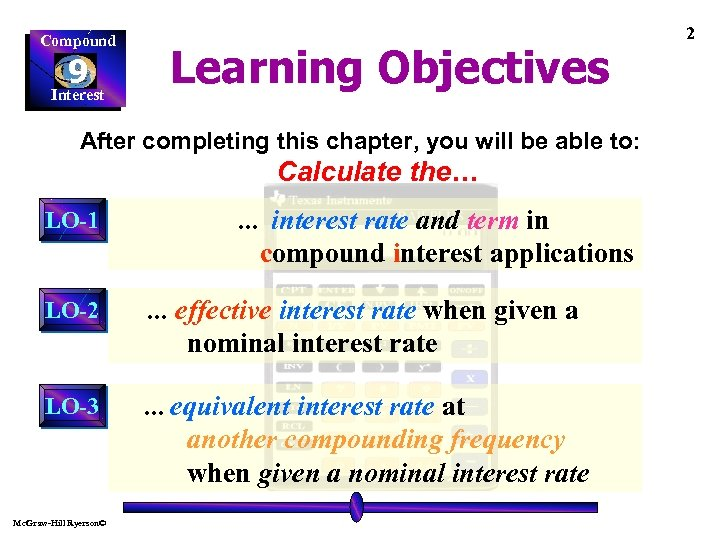Compound Learning Objectives 9 Interest After completing this chapter, you will be able to: Calculate the… LO-1 … interest rate and term in compound interest applications effective interest rate when given a nominal interest rate LO-2 … LO-3 … equivalent Mc. Graw-Hill Ryerson© interest rate at another compounding frequency when given a nominal interest rate 2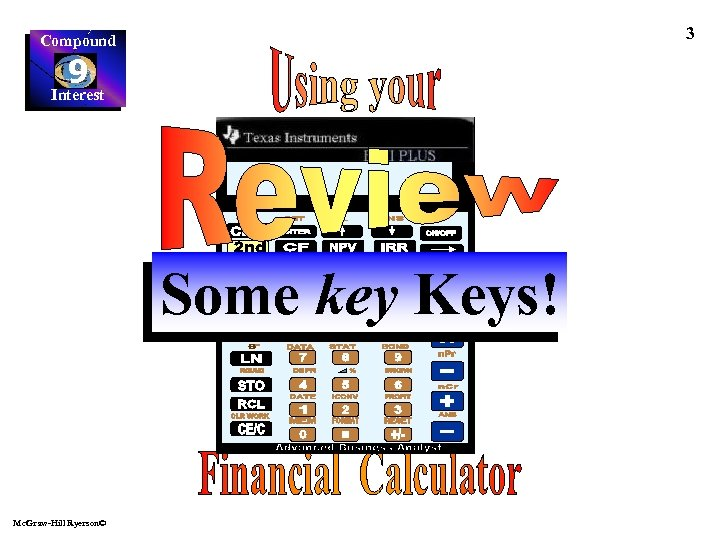3 Compound 9 Interest Some key Keys! Mc. Graw-Hill Ryerson©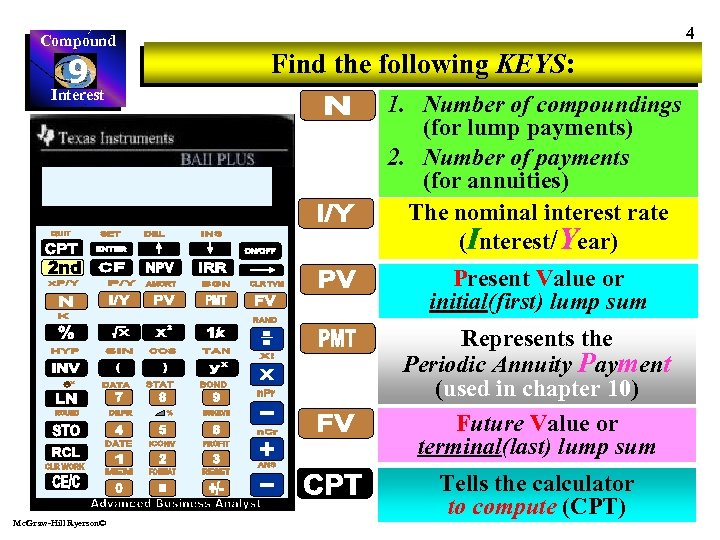Compound 9 Interest 4 Find the following KEYS: 1. Number of compoundings (for lump payments) 2. Number of payments (for annuities) The nominal interest rate (Interest/Year) Present Value or initial(first) lump sum Represents the Periodic Annuity Payment (used in chapter 10) Future Value or terminal(last) lump sum Mc. Graw-Hill Ryerson© Tells the calculator to compute (CPT)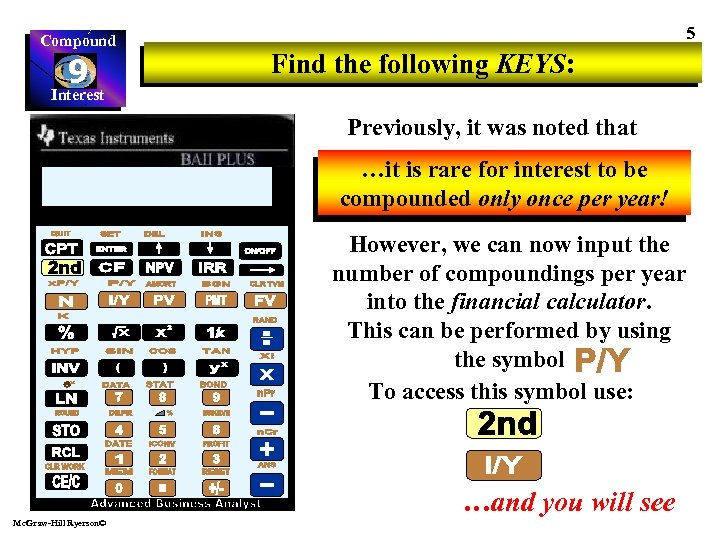Compound 9 Interest 5 Find the following KEYS: Previously, it was noted that …it is rare for interest to be compounded only once per year! However, we can now input the number of compoundings per year into the financial calculator. This can be performed by using the symbol To access this symbol use: …and you will see Mc. Graw-Hill Ryerson©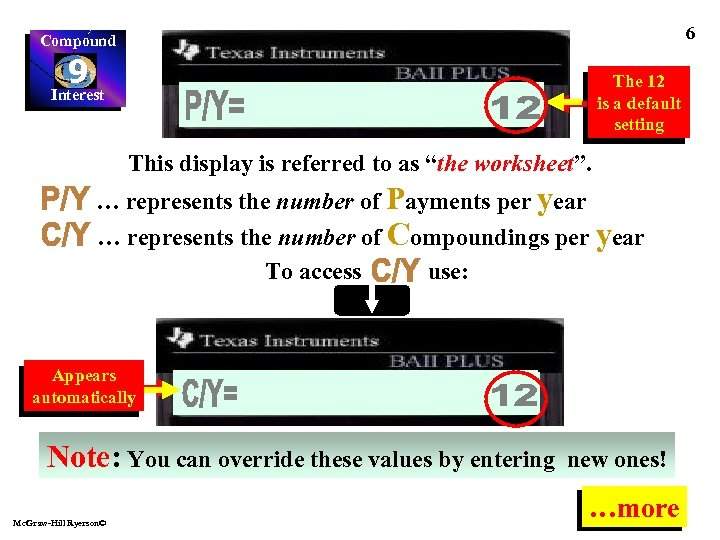6 Compound 9 Interest The 12 is a default setting This display is referred to as “the worksheet”. … represents the number of Payments per year … represents the number of Compoundings per year To access use: Appears automatically Note: You can override these values by entering Mc. Graw-Hill Ryerson© new ones! …more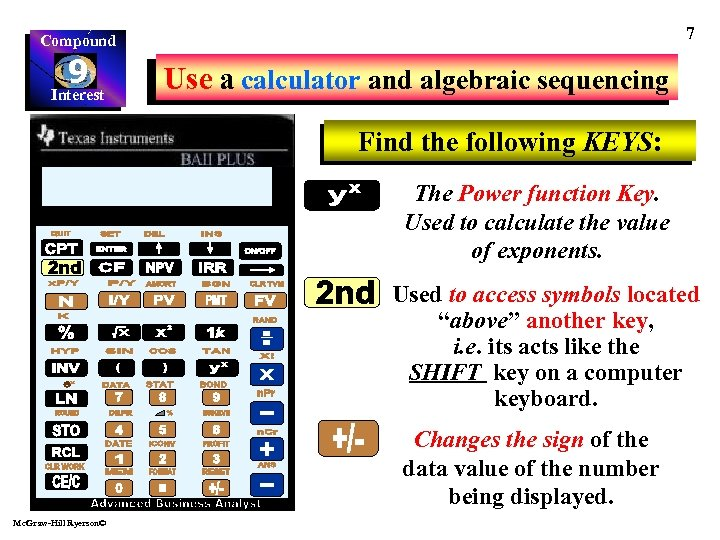7 Compound 9 Interest Use a calculator and algebraic sequencing Find the following KEYS: The Power function Key. Used to calculate the value of exponents. Used to access symbols located “above” another key, i. e. its acts like the SHIFT key on a computer keyboard. Changes the sign of the data value of the number being displayed. Mc. Graw-Hill Ryerson©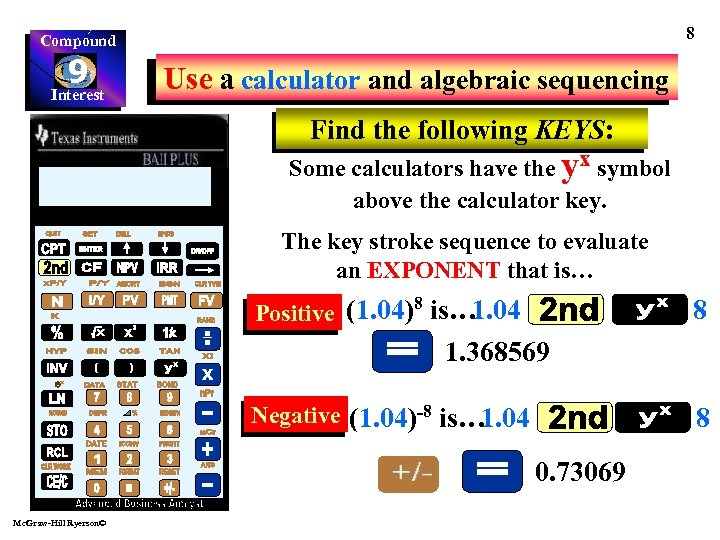8 Compound 9 Interest Use a calculator and algebraic sequencing Find the following KEYS: Some calculators have the yx symbol above the calculator key. The key stroke sequence to evaluate an EXPONENT that is… 8 Positive (1. 04) is… 1. 04 8 1. 368569 Negative (1. 04)-8 is… 1. 04 8 0. 73069 Mc. Graw-Hill Ryerson©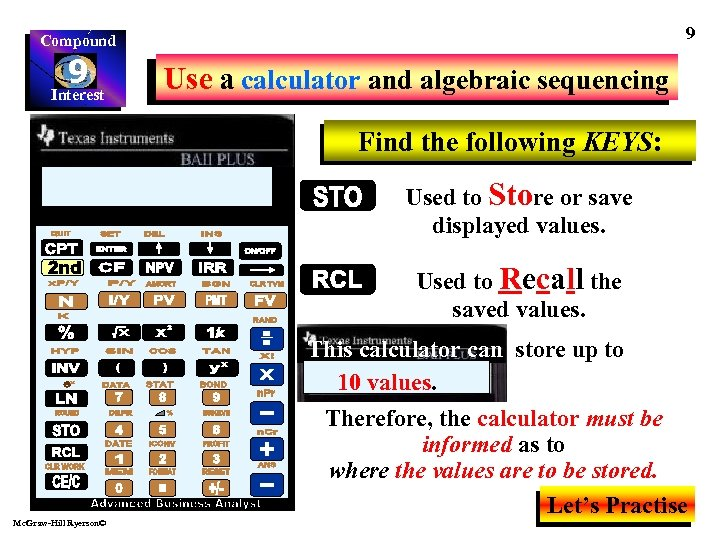9 Compound 9 Interest Use a calculator and algebraic sequencing Find the following KEYS: Used to Store or save displayed values. Used to Recall the saved values. This calculator can store up to 10 values. Mc. Graw-Hill Ryerson© Therefore, the calculator must be informed as to where the values are to be stored. Let’s Practise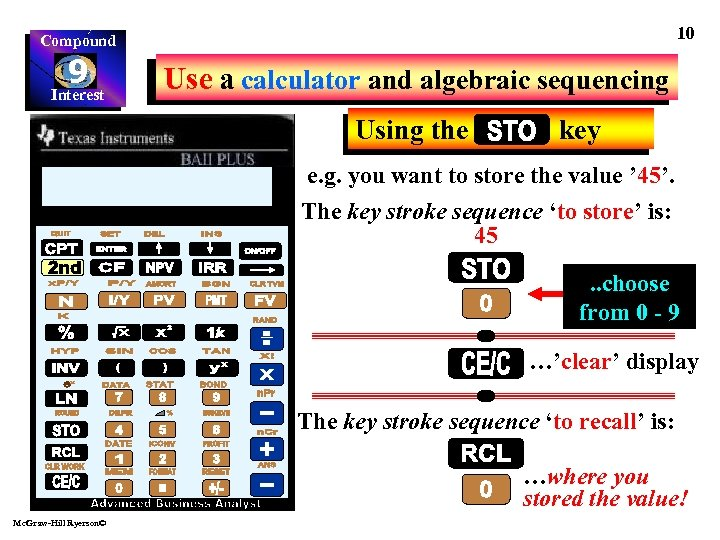10 Compound 9 Interest Use a calculator and algebraic sequencing Using the key e. g. you want to store the value ’ 45’. The key stroke sequence ‘to store’ is: 45. . choose from 0 - 9 …’clear’ display The key stroke sequence ‘to recall’ is: …where you stored the value! Mc. Graw-Hill Ryerson©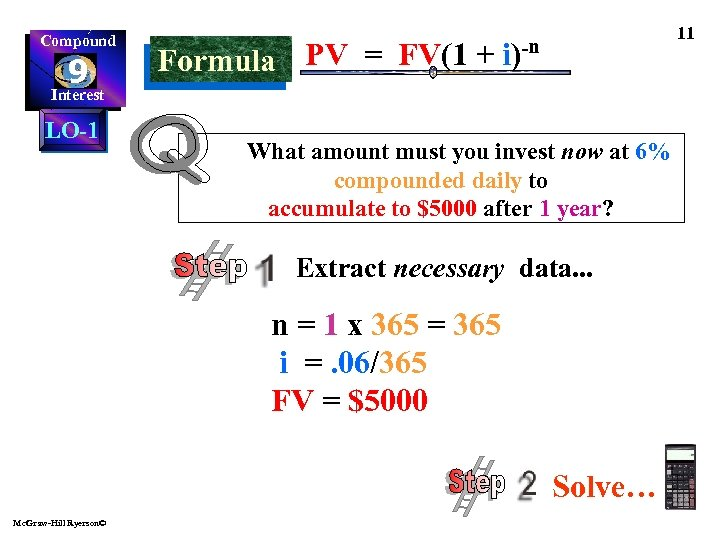Compound 9 Interest LO-1 11 PV = FV(1 + i)-n Formula What amount must you invest now at 6% compounded daily to accumulate to \$5000 after 1 year? Extract necessary data. . . n = 1 x 365 = 365 i =. 06/365 FV = \$5000 Solve… Mc. Graw-Hill Ryerson©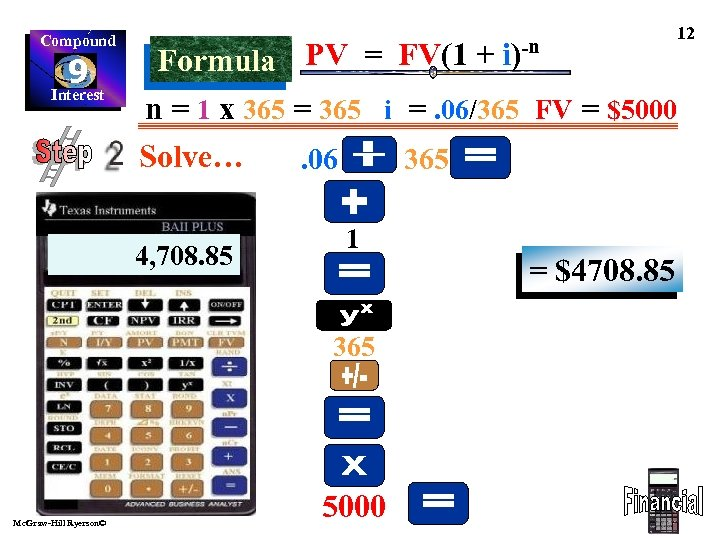Compound 9 Interest 12 PV = FV(1 + i)-n Formula n = 1 x 365 = 365 i =. 06/365 FV = \$5000 Solve… 4, 708. 85 . 06 365 1 365 Mc. Graw-Hill Ryerson© 5000 = \$4708. 85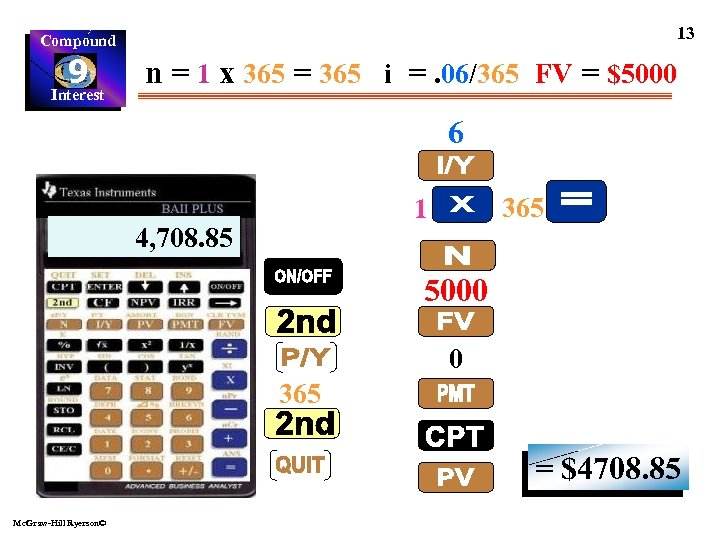13 Compound 9 Interest n = 1 x 365 = 365 i =. 06/365 FV = \$5000 6 365 1 4, 708. 85 5000 0 365 = \$4708. 85 Mc. Graw-Hill Ryerson©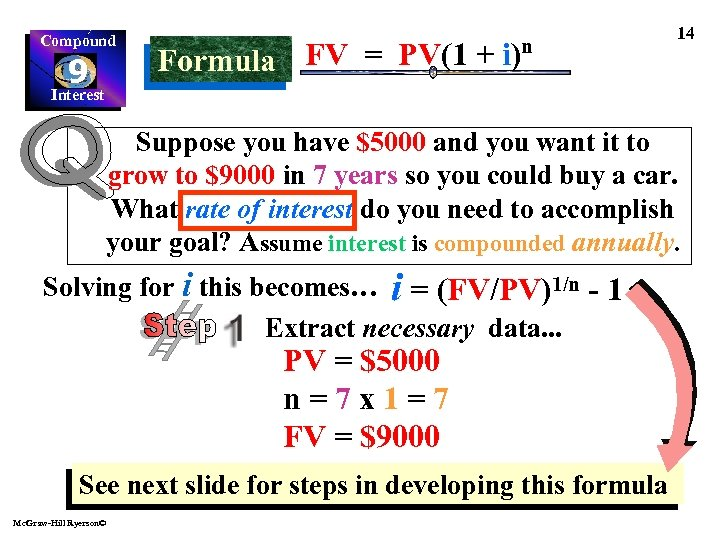Compound 9 Interest FV = PV(1 + i)n Formula 14 Suppose you have \$5000 and you want it to grow to \$9000 in 7 years so you could buy a car. What rate of interest do you need to accomplish your goal? Assume interest is compounded annually. Solving for i this becomes… i = (FV/PV)1/n - 1 Extract necessary data. . . PV = \$5000 n=7 x 1=7 FV = \$9000 See next slide for steps in developing this formula Mc. Graw-Hill Ryerson©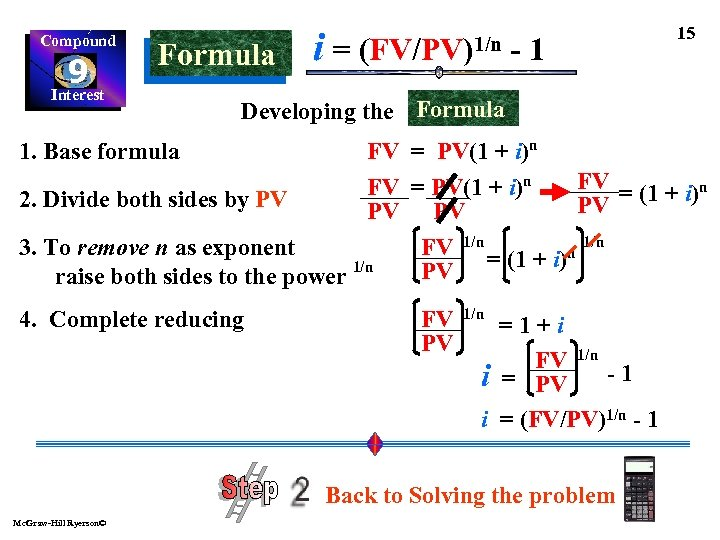Compound 9 Interest Formula i= (FV/PV)1/n -1 15 Developing the Formula FV = PV(1 + i)n 2. Divide both sides by PV PV 1/n FV 1/n 3. To remove n as exponent n 1/n PV = (1 + i) raise both sides to the power 1. Base formula 4. Complete reducing FV PV 1/n =1+i FV 1/n i = PV - 1 i = (FV/PV)1/n - 1 Back to Solving the problem Mc. Graw-Hill Ryerson©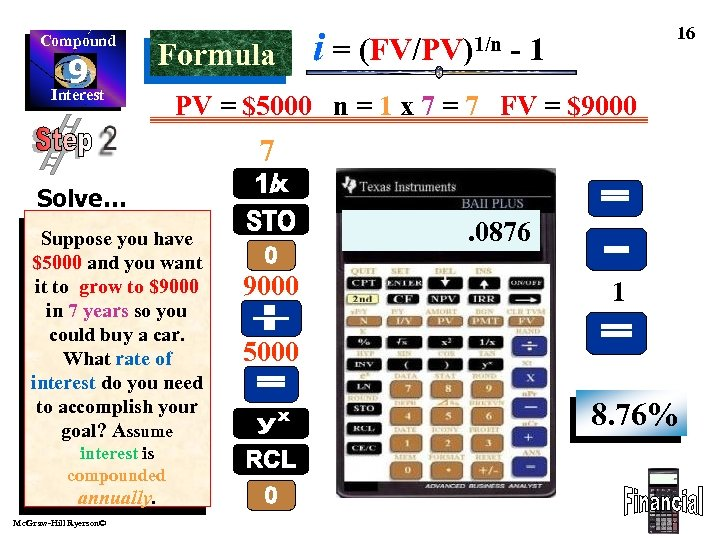Compound 9 Interest Formula i= (FV/PV)1/n 16 -1 PV = \$5000 n = 1 x 7 = 7 FV = \$9000 7 Solve… Suppose you have \$5000 and you want it to grow to \$9000 in 7 years so you could buy a car. What rate of interest do you need to accomplish your goal? Assume interest is compounded annually. Mc. Graw-Hill Ryerson© 1. 0876 9000 1 5000 8. 76%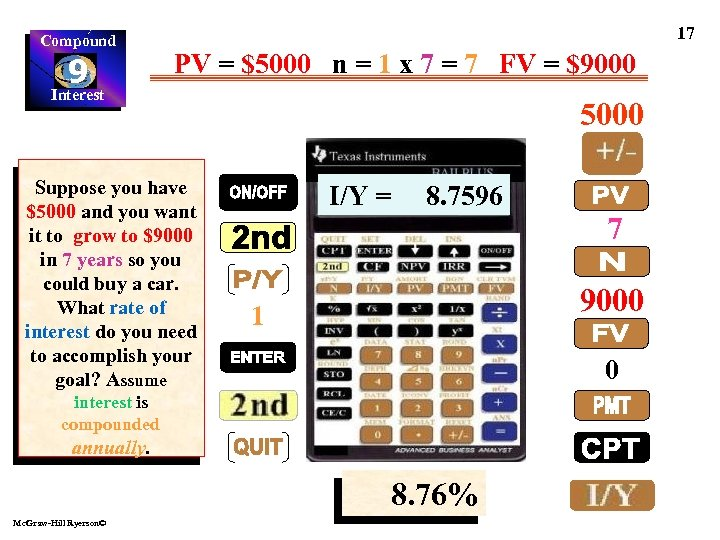Compound 9 Interest 17 PV = \$5000 n = 1 x 7 = 7 FV = \$9000 Suppose you have \$5000 and you want it to grow to \$9000 in 7 years so you could buy a car. What rate of interest do you need to accomplish your goal? Assume 5000 I/Y = 8. 7596 9000 1 0 interest is compounded annually. 8. 76% Mc. Graw-Hill Ryerson© 7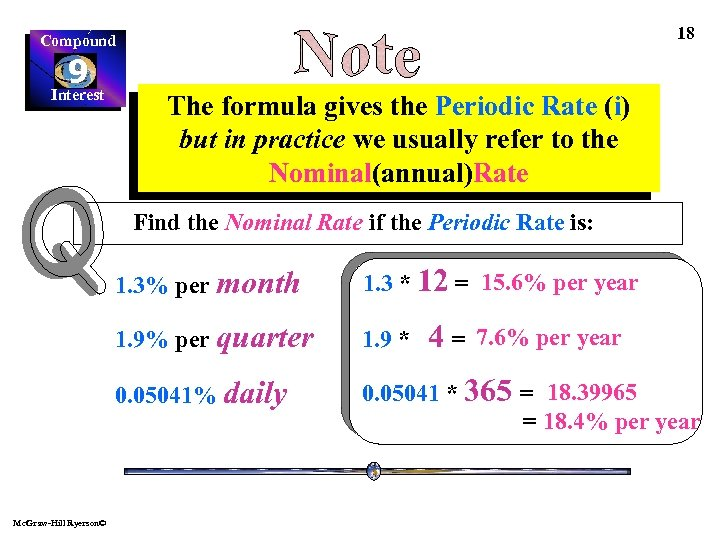18 Compound 9 Interest The formula gives the Periodic Rate (i) but in practice we usually refer to the Nominal(annual)Rate Find the Nominal Rate if the Periodic Rate is: 1. 3% per month 1. 9% per quarter 1. 9 * 0. 05041% daily Mc. Graw-Hill Ryerson© 1. 3 * 12 = 15. 6% per year 0. 05041 * 365 = 18. 39965 = 18. 4% per year 4= 7. 6% per year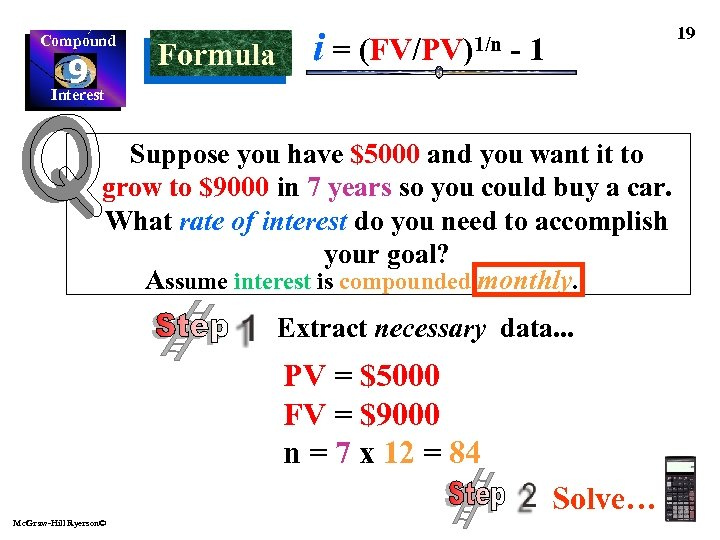Compound 9 Interest Formula i= (FV/PV)1/n 19 -1 Suppose you have \$5000 and you want it to grow to \$9000 in 7 years so you could buy a car. What rate of interest do you need to accomplish your goal? Assume interest is compounded monthly. Extract necessary data. . . PV = \$5000 FV = \$9000 n = 7 x 12 = 84 Solve… Mc. Graw-Hill Ryerson©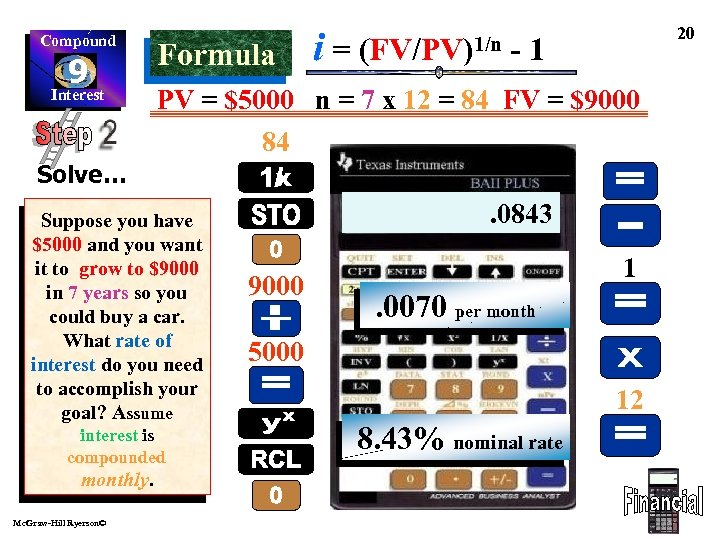Compound 9 Interest Formula i= (FV/PV)1/n 20 -1 PV = \$5000 n = 7 x 12 = 84 FV = \$9000 84 Solve… Suppose you have \$5000 and you want it to grow to \$9000 in 7 years so you could buy a car. What rate of interest do you need to accomplish your goal? Assume interest is compounded monthly. Mc. Graw-Hill Ryerson© 1. 0070. 0843 9000 1 . 0070 per month 5000 12 8. 43% nominal rate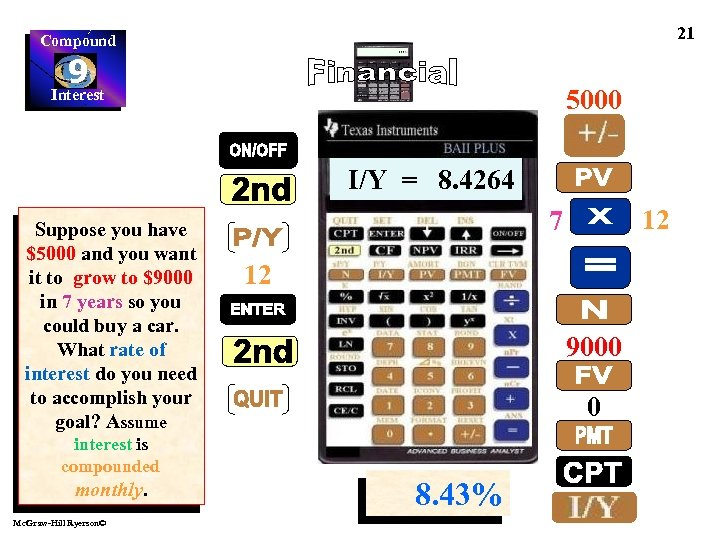21 Compound 9 Interest 5000 I/Y = 8. 4264 Suppose you have \$5000 and you want it to grow to \$9000 in 7 years so you could buy a car. What rate of interest do you need to accomplish your goal? Assume interest is compounded monthly. Mc. Graw-Hill Ryerson© 12 7 12 9000 0 8. 43%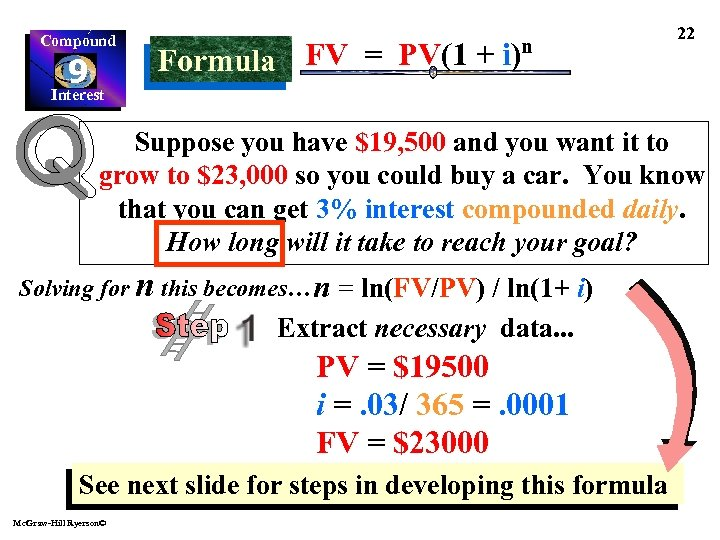Compound 9 Interest FV = PV(1 + i)n Formula 22 Suppose you have \$19, 500 and you want it to grow to \$23, 000 so you could buy a car. You know that you can get 3% interest compounded daily. How long will it take to reach your goal? Solving for n this becomes… n = ln(FV/PV) / ln(1+ i) Extract necessary data. . . PV = \$19500 i =. 03/ 365 =. 0001 FV = \$23000 See next slide for steps in developing this formula Mc. Graw-Hill Ryerson©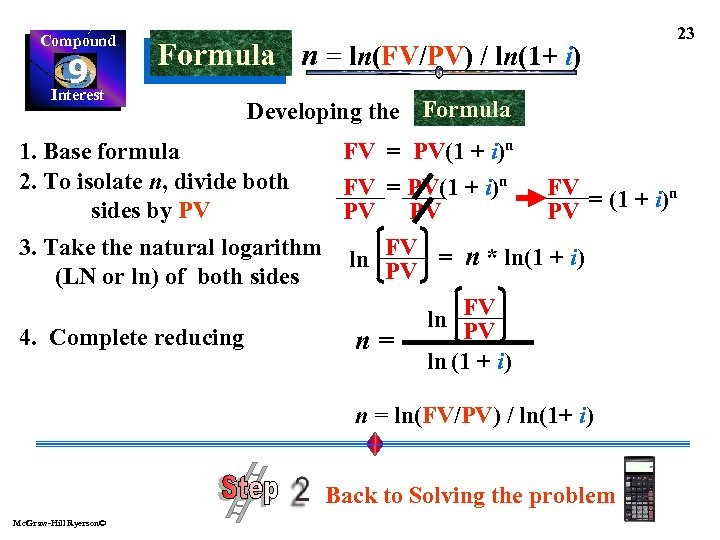Compound 9 Interest Formula n = ln(FV/PV) / ln(1+ i) 23 Developing the Formula FV = PV(1 + i)n FV = (1 + i)n PV PV PV 3. Take the natural logarithm ln FV = n * ln(1 + i) PV (LN or ln) of both sides FV ln PV 4. Complete reducing n= ln (1 + i) 1. Base formula 2. To isolate n, divide both sides by PV n = ln(FV/PV) / ln(1+ i) Back to Solving the problem Mc. Graw-Hill Ryerson©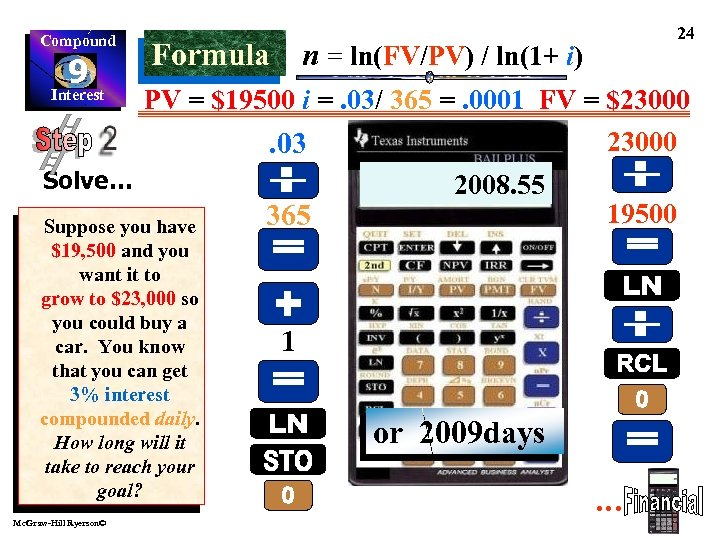Compound 9 Interest n = ln(FV/PV) / ln(1+ i) Formula PV = \$19500 i =. 03/ 365 =. 0001 FV = \$23000. 03 Solve… Suppose you have \$19, 500 and you want it to grow to \$23, 000 so you could buy a car. You know that you can get 3% interest compounded daily. How long will it take to reach your goal? Mc. Graw-Hill Ryerson© 24 365 0. 1651 0. 0001 2008. 55 19500 1 or 2009 days …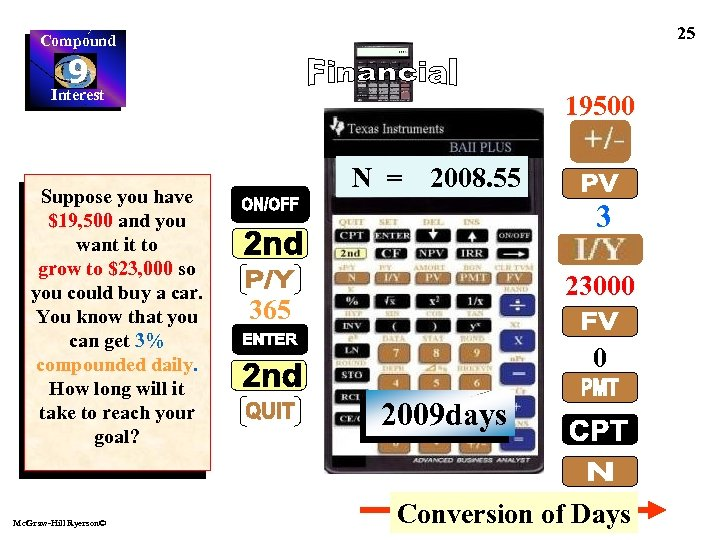25 Compound 9 Interest Suppose you have \$19, 500 and you want it to grow to \$23, 000 so you could buy a car. You know that you can get 3% compounded daily. How long will it take to reach your goal? Mc. Graw-Hill Ryerson© 19500 N = 2008. 55 3 23000 365 0 2009 days Conversion of Days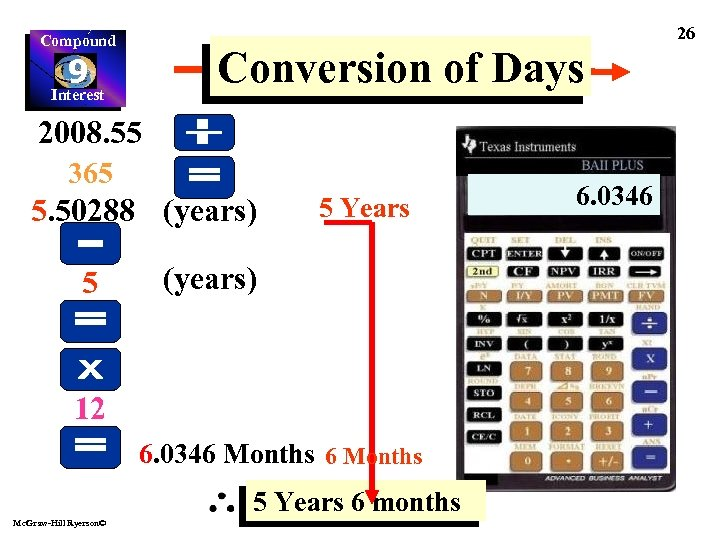26 Compound Conversion of Days 9 Interest 2008. 55 365 5. 50288 (years) 5 5 Years (years) 12 6. 0346 Months 5 Years 6 months Mc. Graw-Hill Ryerson© 0. 50288 5. 50288 2008. 55 6. 0346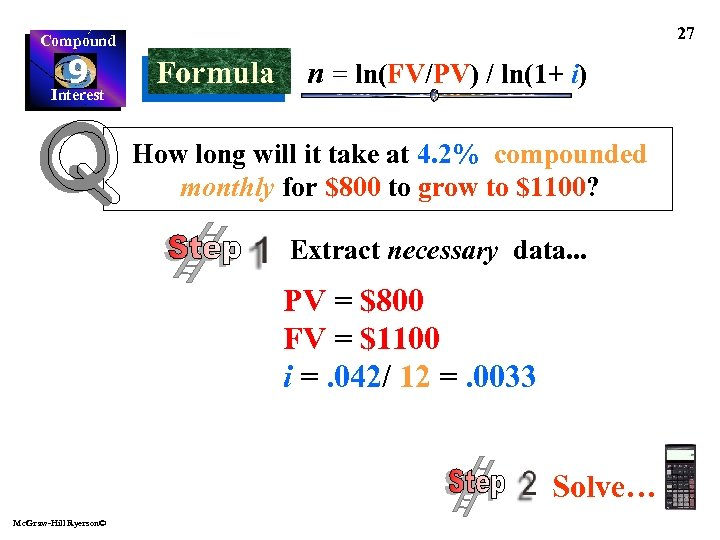27 Compound 9 Interest Formula n = ln(FV/PV) / ln(1+ i) How long will it take at 4. 2% compounded monthly for \$800 to grow to \$1100? Extract necessary data. . . PV = \$800 FV = \$1100 i =. 042/ 12 =. 0033 Solve… Mc. Graw-Hill Ryerson©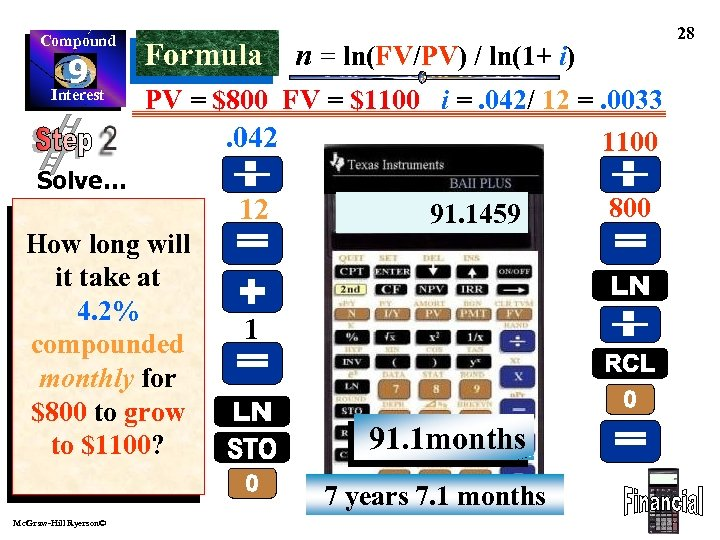Compound 9 Interest Formula n = ln(FV/PV) / ln(1+ i) PV = \$800 FV = \$1100 i =. 042/ 12 =. 0033. 042 1100 Solve… How long will it take at 4. 2% compounded monthly for \$800 to grow to \$1100? 12 91. 1459 0. 0035. 3185 1 91. 1 months 7 years 7. 1 months Mc. Graw-Hill Ryerson© 28 800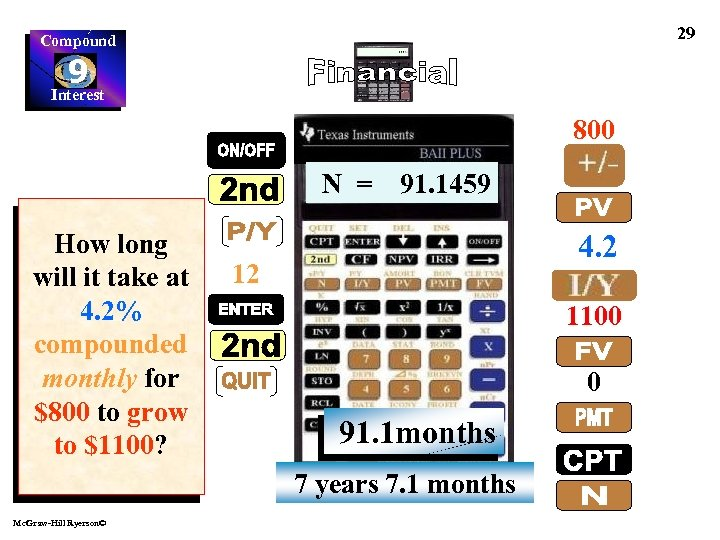29 Compound 9 Interest 800 N = How long will it take at 4. 2% compounded monthly for \$800 to grow to \$1100? 91. 1459 4. 2 12 1100 0 91. 1 months 7 years 7. 1 months Mc. Graw-Hill Ryerson©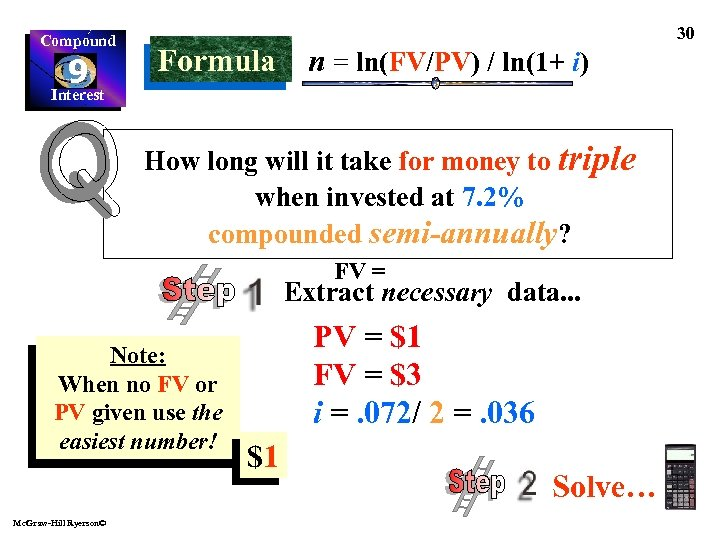Compound 9 Interest Formula n = ln(FV/PV) / ln(1+ i) How long will it take for money to triple when invested at 7. 2% compounded semi-annually? FV = Extract necessary data. . . Note: When no FV or PV given use the easiest number! Mc. Graw-Hill Ryerson© PV = \$1 FV = \$3 i =. 072/ 2 =. 036 \$1 Solve… 30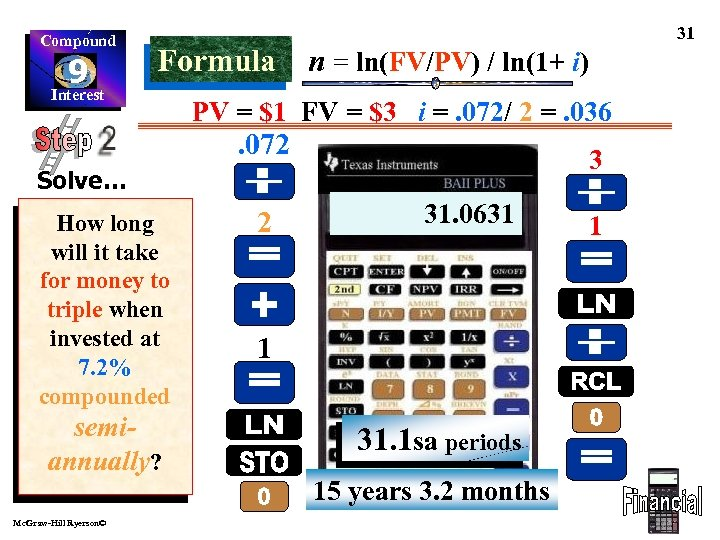Compound 9 Interest Formula n = ln(FV/PV) / ln(1+ i) PV = \$1 FV = \$3 i =. 072/ 2 =. 036 . 072 3 Solve… How long will it take for money to triple when invested at 7. 2% compounded semiannually? Mc. Graw-Hill Ryerson© 31 2 31. 0631 1. 0986 0. 0354 1 31. 1 sa periods 15 years 3. 2 months 1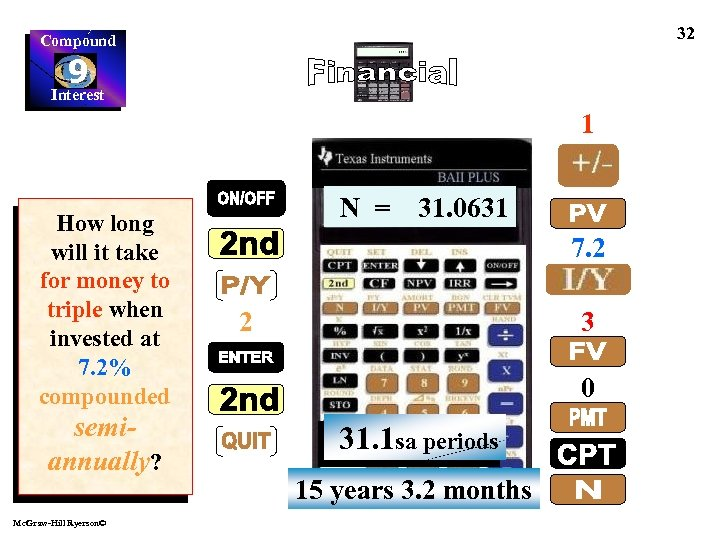32 Compound 9 Interest 1 How long will it take for money to triple when invested at 7. 2% compounded semiannually? Mc. Graw-Hill Ryerson© N = 31. 0631 7. 2 2 3 0 31. 1 sa periods 15 years 3. 2 months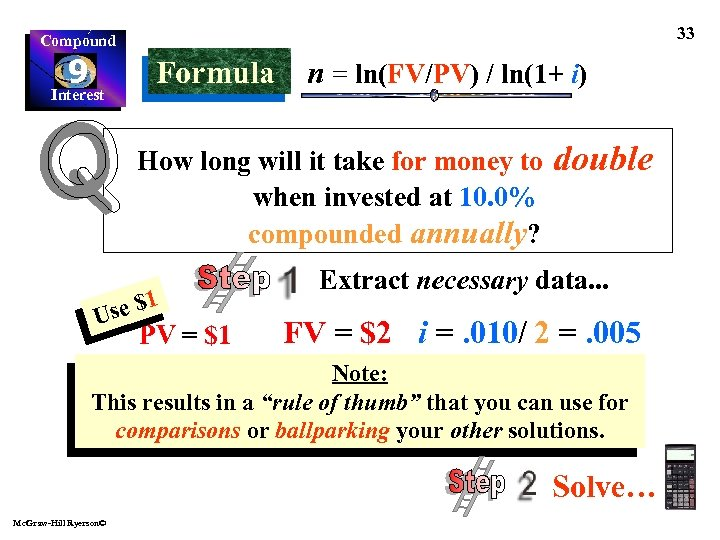33 Compound 9 Interest Formula n = ln(FV/PV) / ln(1+ i) How long will it take for money to when invested at 10. 0% compounded annually? se \$1 U PV = \$1 double Extract necessary data. . . FV = \$2 i =. 010/ 2 =. 005 Note: This results in a “rule of thumb” that you can use for comparisons or ballparking your other solutions. Solve… Mc. Graw-Hill Ryerson©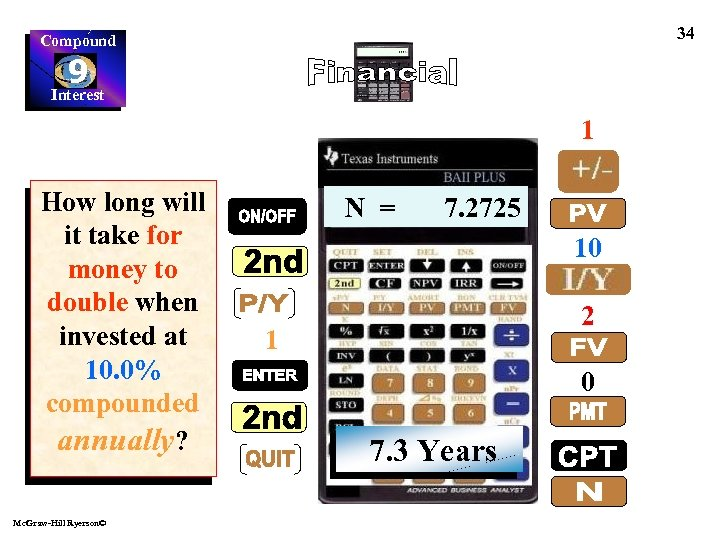34 Compound 9 Interest 1 How long will it take for money to double when invested at 10. 0% compounded annually? Mc. Graw-Hill Ryerson© N = 7. 2725 10 2 1 0 7. 3 Years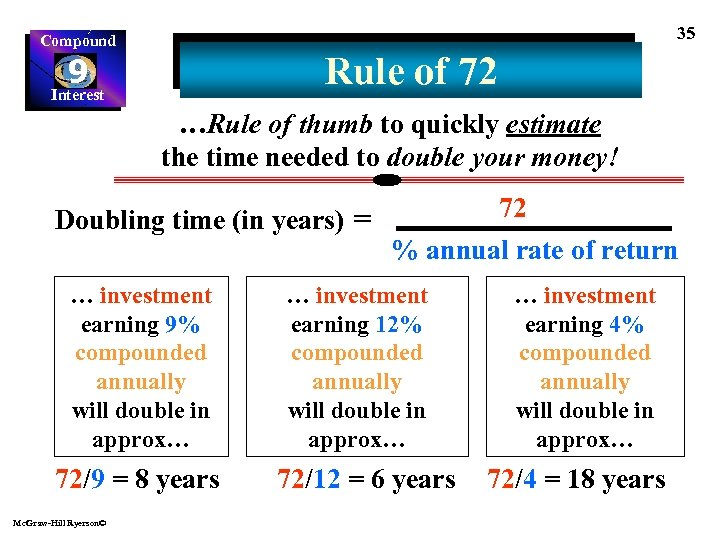35 Compound Rule of 72 9 Interest …Rule of thumb to quickly estimate the time needed to double your money! Doubling time (in years) = … investment earning 9% compounded annually will double in approx… 72/9 = 8 years Mc. Graw-Hill Ryerson© 72 % annual rate of return … investment earning 12% compounded annually will double in approx… 72/12 = 6 years … investment earning 4% compounded annually will double in approx… 72/4 = 18 years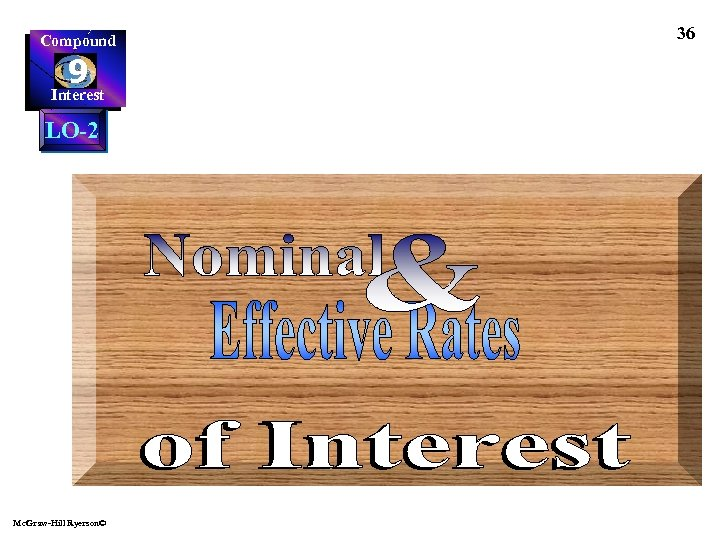Compound 9 Interest LO-2 Mc. Graw-Hill Ryerson© 36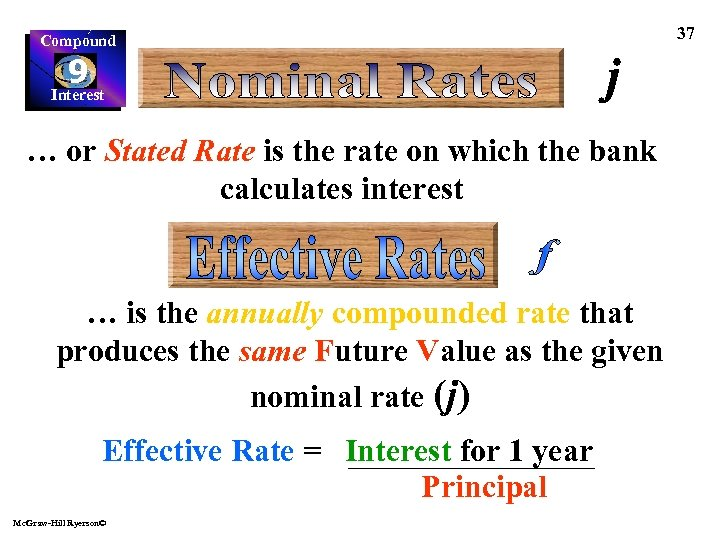Compound 9 Interest … or Stated Rate is the rate on which the bank calculates interest … is the annually compounded rate that produces the same Future Value as the given nominal rate (j) Effective Rate = Interest for 1 year Principal Mc. Graw-Hill Ryerson© 37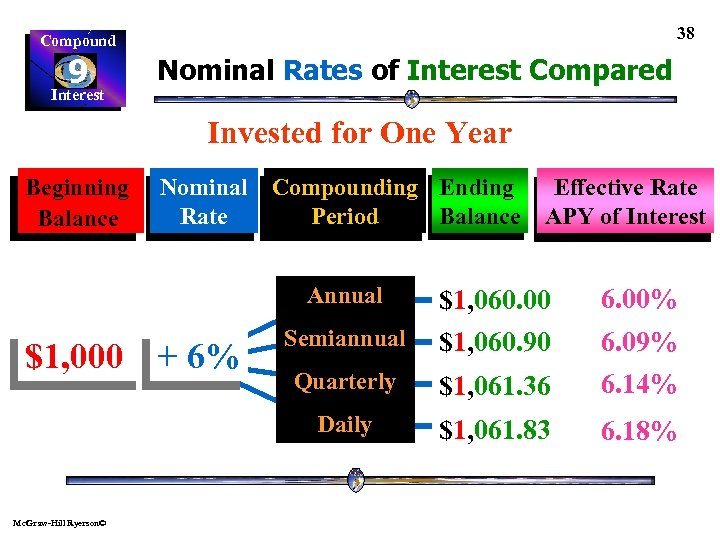38 Compound 9 Interest Nominal Rates of Interest Compared Invested for One Year Beginning Balance Nominal Compounding Ending Rate Period Balance Annual Effective Rate APY of Interest 6. 00% Mc. Graw-Hill Ryerson© Quarterly \$1, 061. 36 6. 09% 6. 14% Daily \$1, 000 + 6% Semiannual \$1, 060. 00 \$1, 060. 90 \$1, 061. 83 6. 18%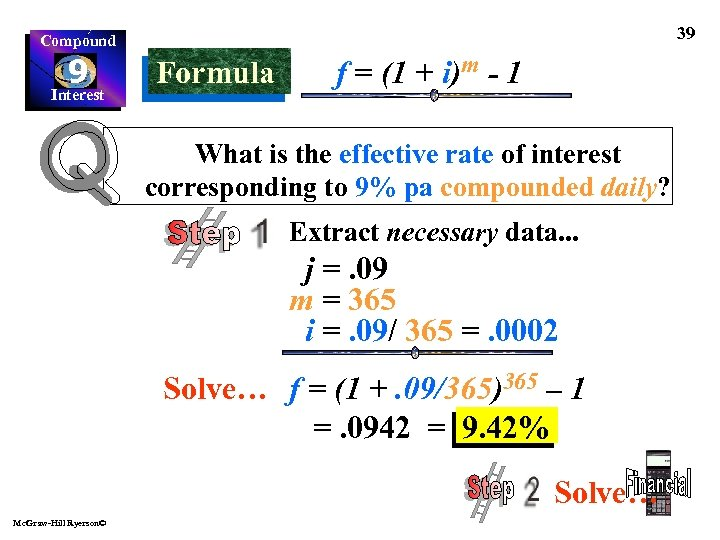39 Compound 9 Interest Formula f = (1 + i)m - 1 What is the effective rate of interest corresponding to 9% pa compounded daily? Extract necessary data. . . j =. 09 m = 365 i =. 09/ 365 =. 0002 Solve… f = (1 +. 09/365)365 – 1 =. 0942 = 9. 42% Solve… Mc. Graw-Hill Ryerson©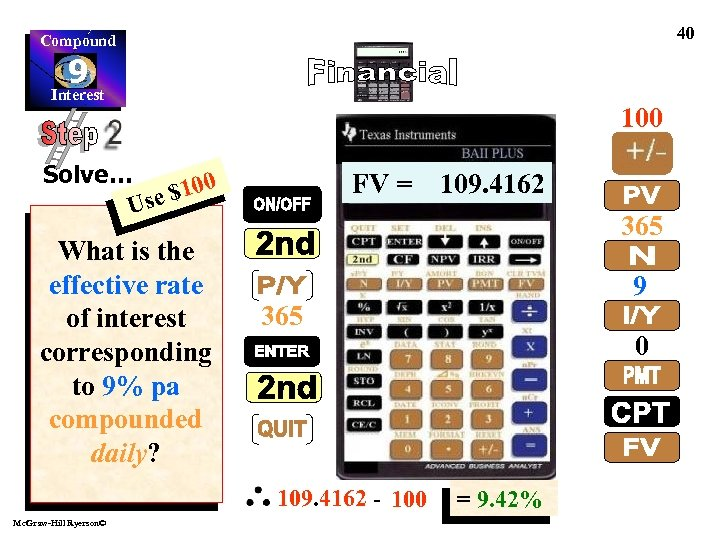40 Compound 9 Interest 100 Solve… \$100 Use What is the effective rate of interest corresponding to 9% pa compounded daily? FV = 109. 4162 365 109. 4162 - 100 Mc. Graw-Hill Ryerson© 9 0 = 9. 42%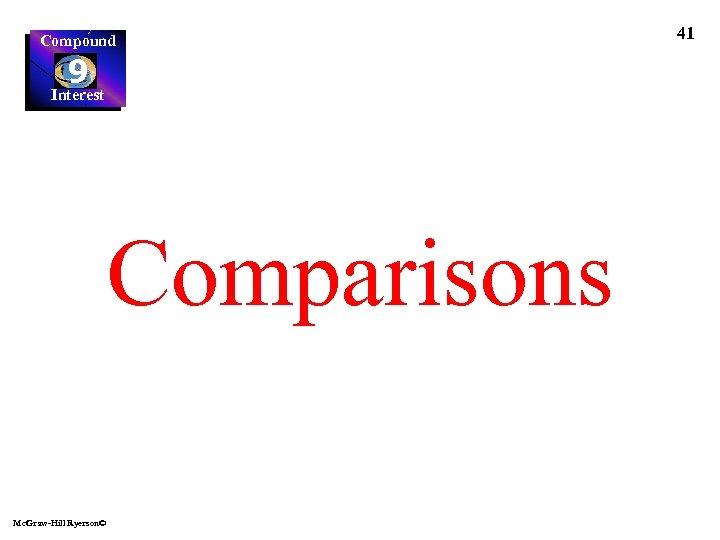Compound 9 Interest Comparisons Mc. Graw-Hill Ryerson© 41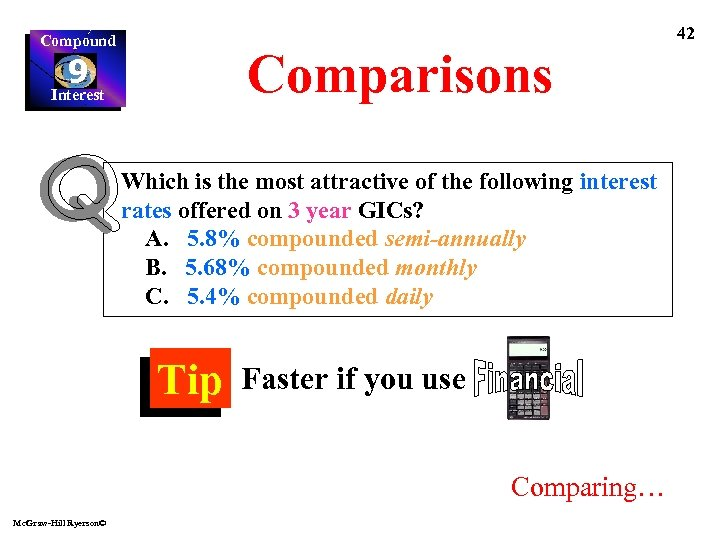42 Compound Comparisons 9 Interest Which is the most attractive of the following interest rates offered on 3 year GICs? A. 5. 8% compounded semi-annually B. 5. 68% compounded monthly C. 5. 4% compounded daily Tip Faster if you use Comparing… Mc. Graw-Hill Ryerson©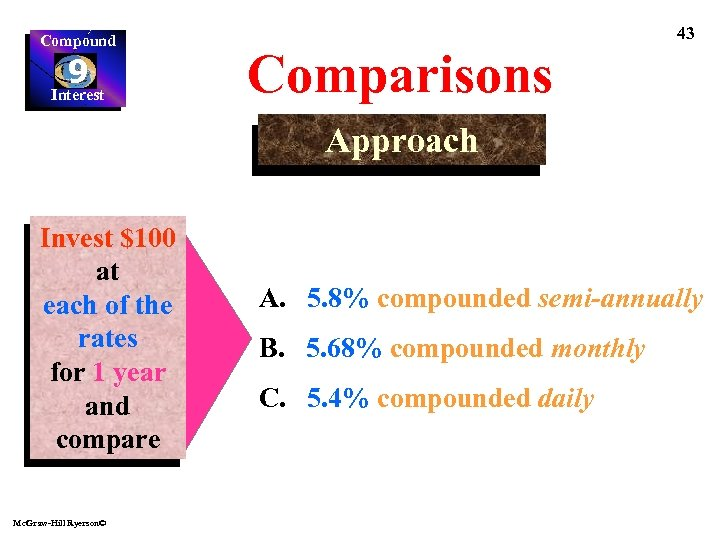Compound 9 Interest 43 Comparisons Approach Invest \$100 at each of the rates for 1 year and compare Mc. Graw-Hill Ryerson© A. 5. 8% compounded semi-annually B. 5. 68% compounded monthly C. 5. 4% compounded daily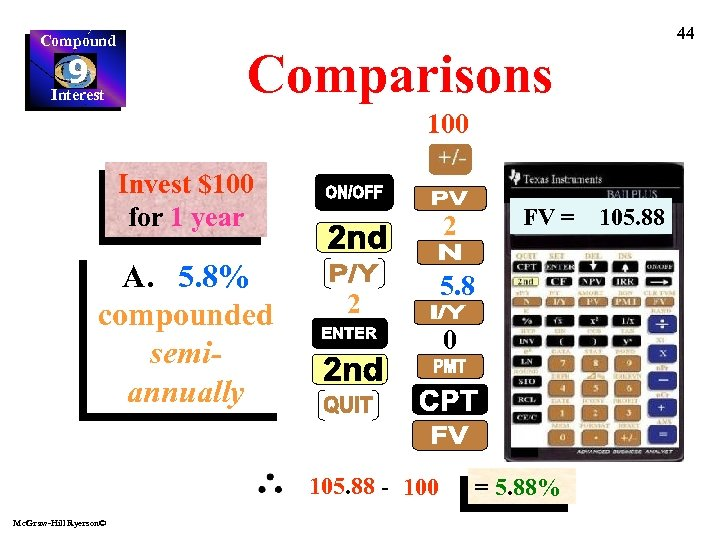Compound 9 Interest 44 Comparisons 100 Invest \$100 for 1 year A. 5. 8% compounded semiannually 2 2 5. 8 0 105. 88 - 100 Mc. Graw-Hill Ryerson© FV = = 5. 88% 105. 88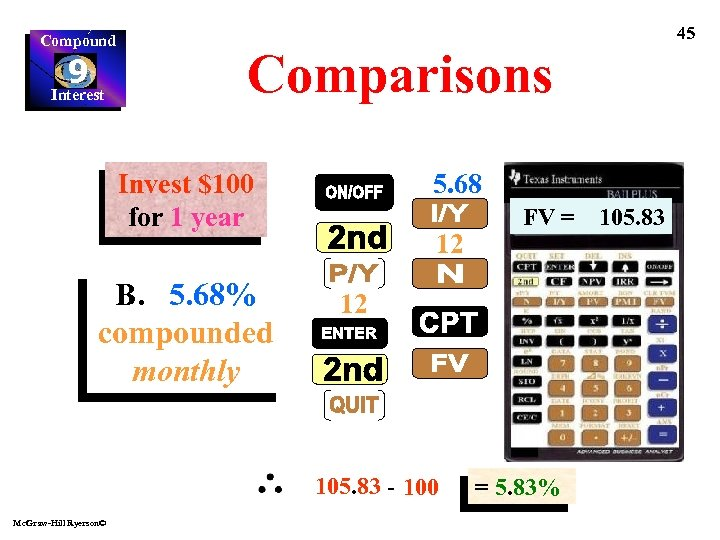Compound 9 Interest 45 Comparisons Invest \$100 for 1 year B. 5. 68% compounded monthly 5. 68 12 12 105. 83 - 100 Mc. Graw-Hill Ryerson© FV = = 5. 83% 105. 83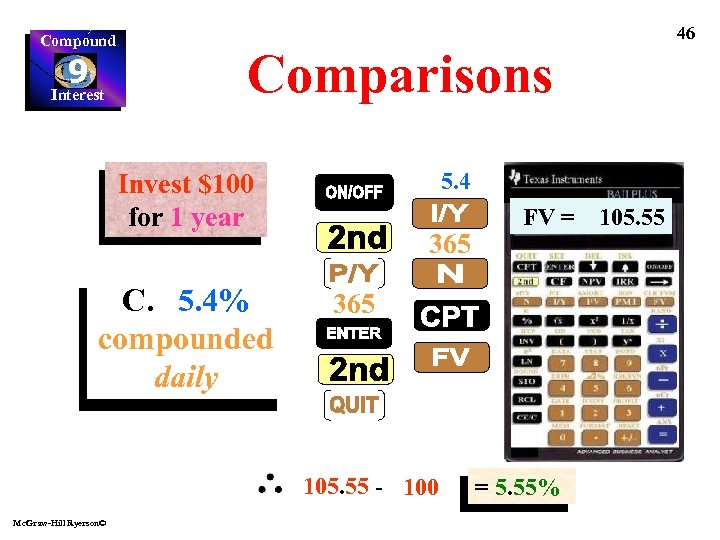Compound 9 Interest 46 Comparisons 5. 4 Invest \$100 for 1 year C. 5. 4% compounded daily 365 105. 55 - 100 Mc. Graw-Hill Ryerson© FV = = 5. 55% 105. 55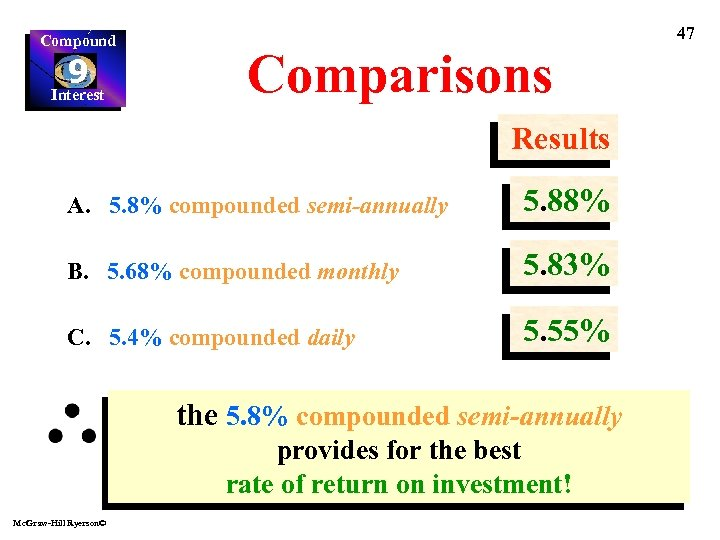Compound 9 Interest 47 Comparisons Results A. 5. 8% compounded semi-annually 5. 88% B. 5. 68% compounded monthly 5. 83% C. 5. 4% compounded daily 5. 55% the 5. 8% compounded semi-annually provides for the best rate of return on investment! Mc. Graw-Hill Ryerson©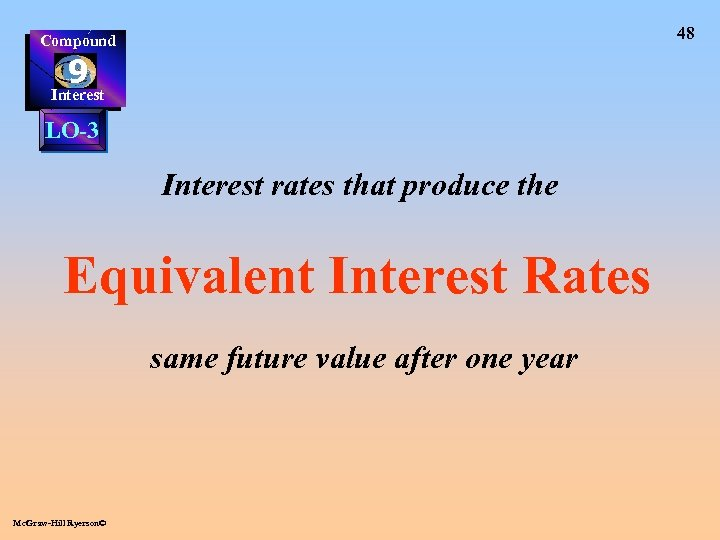48 Compound 9 Interest LO-3 Interest rates that produce the Equivalent Interest Rates same future value after one year Mc. Graw-Hill Ryerson©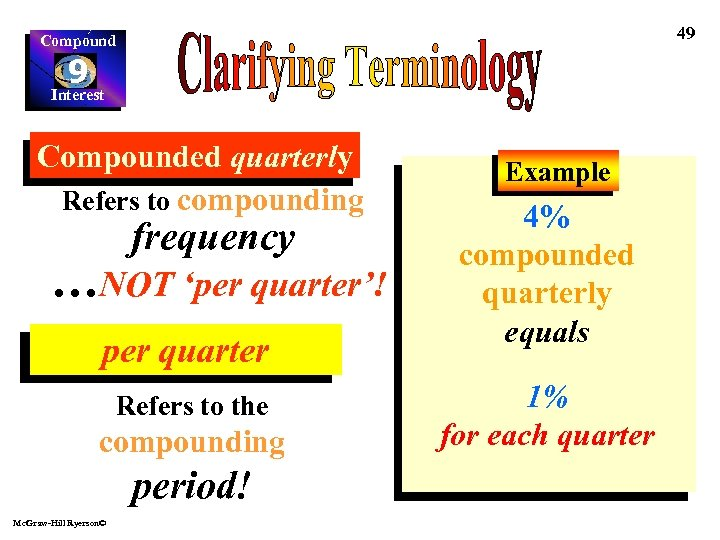49 Compound 9 Interest Compounded quarterly Refers to compounding frequency …NOT ‘per quarter’! per quarter Refers to the compounding period! Mc. Graw-Hill Ryerson© Example 4% compounded quarterly equals 1% for each quarter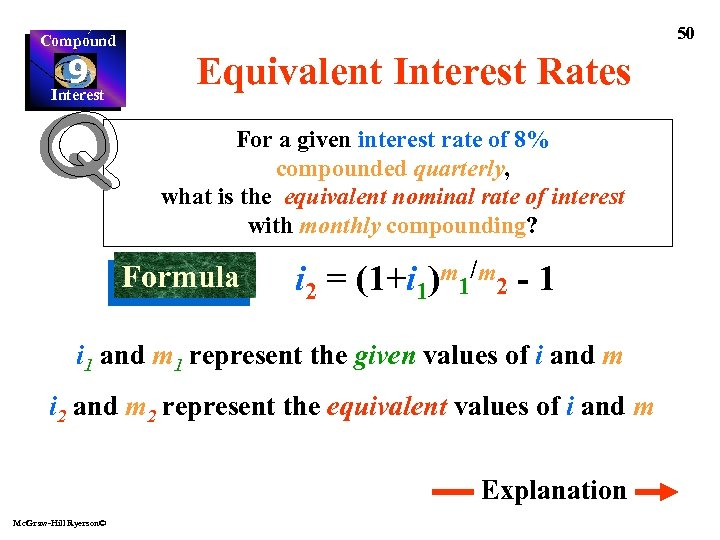Compound 9 Interest 50 Equivalent Interest Rates For a given interest rate of 8% compounded quarterly, what is the equivalent nominal rate of interest with monthly compounding? Formula i 2 = (1+i 1)m 1/m 2 - 1 i 1 and m 1 represent the given values of i and m i 2 and m 2 represent the equivalent values of i and m Explanation Mc. Graw-Hill Ryerson©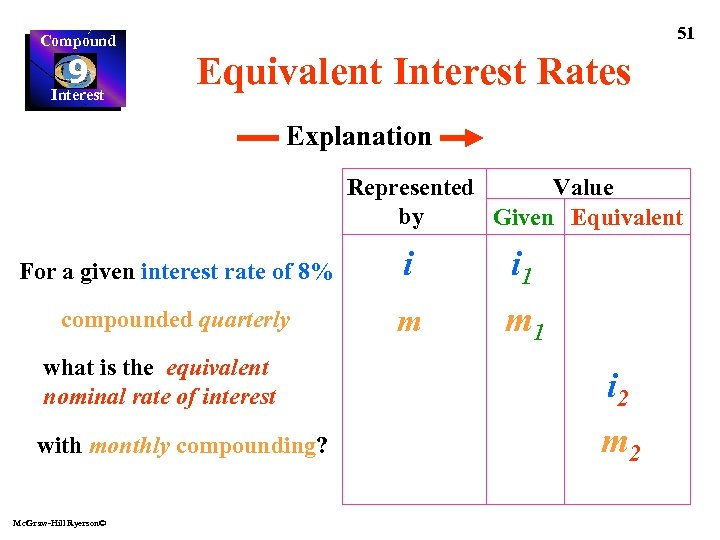Compound 9 Interest 51 Equivalent Interest Rates Explanation Value Represented by Given Equivalent For a given interest rate of 8% i i 1 compounded quarterly m m 1 what is the equivalent nominal rate of interest i 2 with monthly compounding? m 2 Mc. Graw-Hill Ryerson©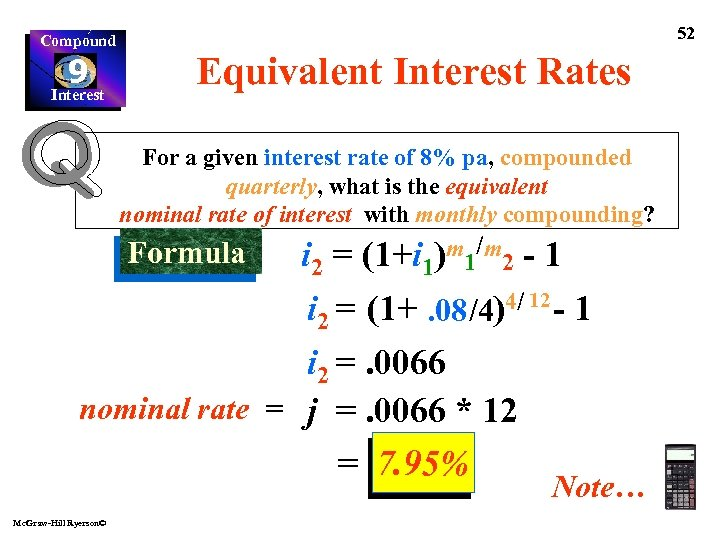Compound 9 Interest 52 Equivalent Interest Rates For a given interest rate of 8% pa, compounded quarterly, what is the equivalent nominal rate of interest with monthly compounding? Formula i 2 = (1+i 1)m 1/m 2 - 1 4/ 12 - 1 i 2 = (1+. 08 /4) i 2 =. 0066 nominal rate = j =. 0066 * 12 = 7. 95% Mc. Graw-Hill Ryerson© Note…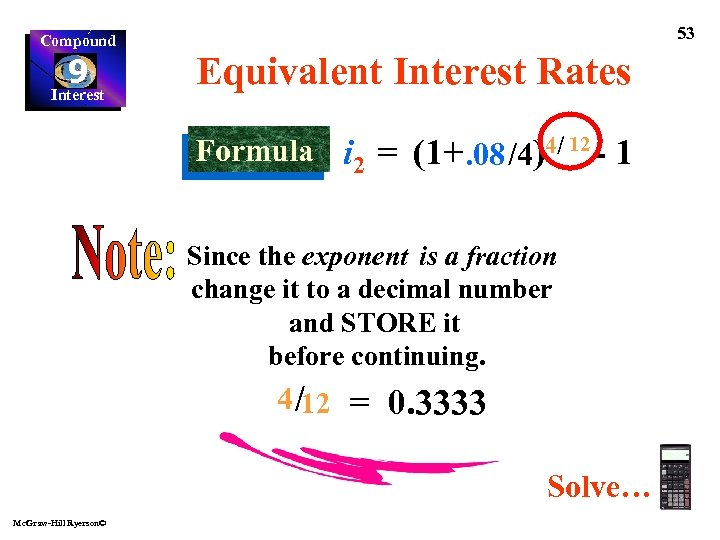Compound 9 Interest 53 Equivalent Interest Rates Formula i 2 = (1+. 08 /4)4/ 12 - 1 Since the exponent is a fraction change it to a decimal number and STORE it before continuing. 4 /12 = 0. 3333 Solve… Mc. Graw-Hill Ryerson©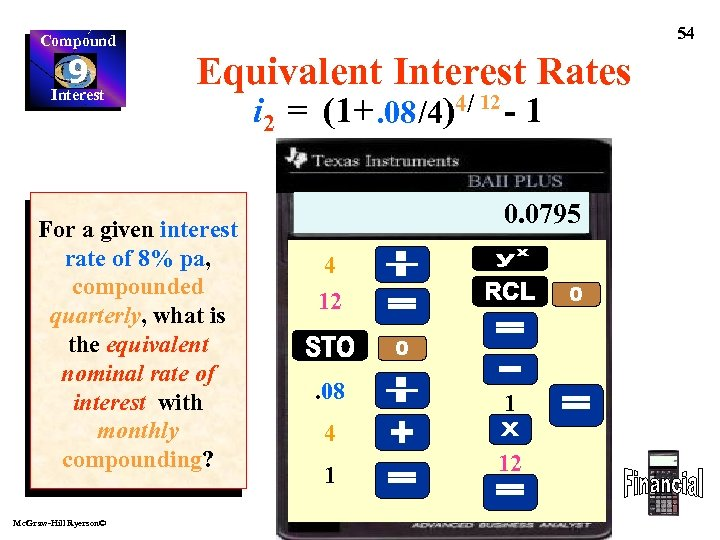Compound 9 Interest 54 Equivalent Interest Rates For a given interest rate of 8% pa, compounded quarterly, what is the equivalent nominal rate of interest with monthly compounding? Mc. Graw-Hill Ryerson© i 2 = (1+. 08 /4)4/ 12 - 1 0. 0066 1. 0066 0. 0795 0. 3333 1. 02 4 12 . 08 1 4 1 12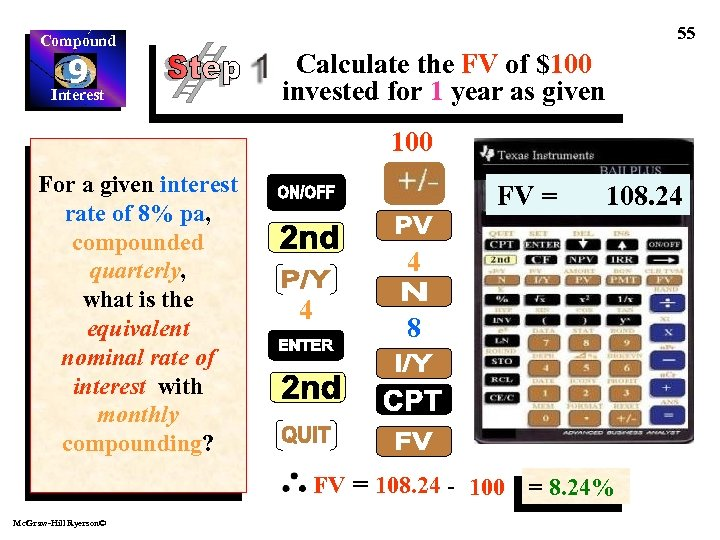Compound 9 Interest 55 Calculate the FV of \$100 invested for 1 year as given 100 For a given interest rate of 8% pa, compounded quarterly, what is the equivalent nominal rate of interest with monthly compounding? FV = 4 4 8 FV = 108. 24 - 100 Mc. Graw-Hill Ryerson© 108. 24 = 8. 24%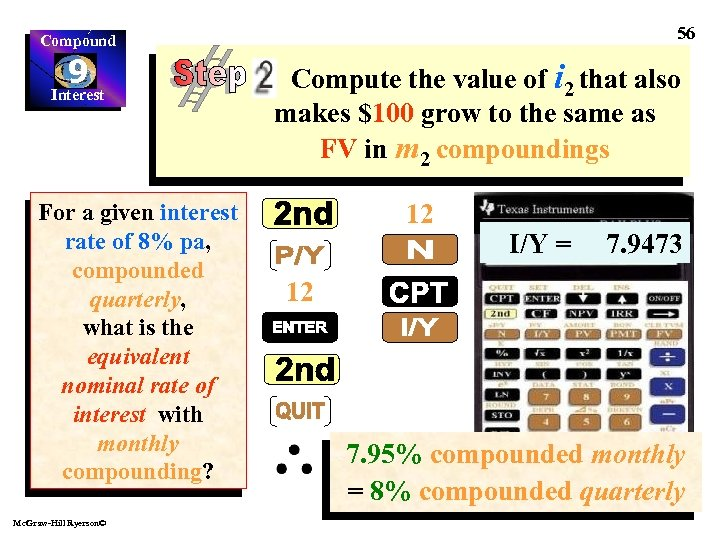56 Compound 9 Interest For a given interest rate of 8% pa, compounded quarterly, what is the equivalent nominal rate of interest with monthly compounding? Mc. Graw-Hill Ryerson© Compute the value of i 2 that also makes \$100 grow to the same as FV in m 2 compoundings 12 I/Y = 7. 9473 12 7. 95% compounded monthly = 8% compounded quarterlyCompound 9 Interest This completes Chapter 9 Mc. Graw-Hill Ryerson© 57